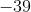# High School Math : Conic Sections

## Example Questions

### Example Question #1 : Pre Calculus

What is the center and radius of the circle indicated by the equation?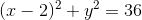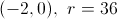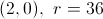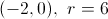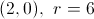Explanation:

A circle is defined by an equation in the format.

The center is indicated by the point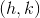and the radius.

In the equation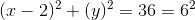, the center is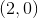and the radius is.

### Example Question #1 : Conic Sections

What is the shape of the graph indicated by the equation?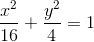Hyperbola

Parabola

Ellipse

Circle

Ellipse

Explanation:

An ellipse has an equation that can be written in the format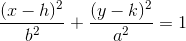. The center is indicated by, or in this case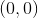.

### Example Question #3 : Pre Calculus

A conic section is represented by the following equation: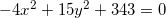What type of conic section does this equation represent?

Parabola

Ellipse

Hyperbola

Circle

Hyperbola

Explanation:

The simplest way to know what kind of conic section an equation represents is by checking the coefficients in front of each variable. The equation must be in general form while you do this check. Luckily, this equation is already in general form, so it's easy to see. The general equation for a conic section is the following: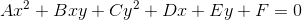Assuming the term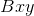is 0 (which it usually is):

• If A equals C, the equation is a circle.
• If A and C have the same sign (but are not equal to each other), the equation is an ellipse.
• If either A or C equals 0, the equation is a parabola.
• If A and C are different signs (i.e. one is negative and one is positive), the equation is a hyperbola.

### Example Question #4 : Pre Calculus

A conic section is represented by the following equation: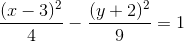Which of the following best describes this equation?

vertical ellipse with center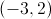and a major axis length of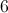vertical parabola with vertexand a vertical stretch factor ofhorizontal hyperbola with center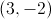and asymptotes with slopes ofand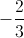horizontal hyperbola with center ofand asymptotes with slopes of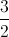and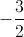vertical hyperbola with centerand asymptotes with slopes ofandhorizontal hyperbola with center ofand asymptotes with slopes ofandExplanation:

First, we need to make sure the conic section equation is in a form we recognize. Luckily, this equation is already in standard form: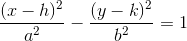The first step is to determine the type of conic section this equation represents. Because there are two squared variables (and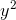), this equation cannot be a parabola.  Because the coefficients in front of the squared variables are different signs (i.e. one is negative and the other is positive), this equation must be a hyperbola, not an ellipse.

In a hyperbola, the squared term with a positive coefficient represents the direction in which the hyperbola opens. In other words, if theterm is positive, the hyperbola opens horizontally. If theterm is positive, the hyperbola opens vertically. Therefore, this is a horizontal hyperbola.

The center is always found at, which in this case is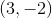.

That leaves only the asymptotes. For a hyperbola, the slopes of the asymptotes can be found by dividingby(remember to always put the vertical value,, above the horizontal value,). Remember that these slopes always come in pairs, with one being positive and the other being negative.

In this case,is 3 andis 2, so we get slopes ofand.

### Example Question #5 : Pre Calculus

Find the vertex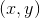for a parabola with equation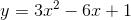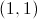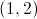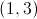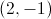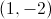Explanation:

For any parabola of the form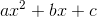,  the-coordinate of its vertex is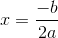So here, we have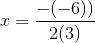We plug this back into the original equation to find: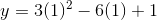### Example Question #6 : Pre Calculus

What is the minimal value of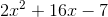over all real numbers?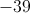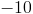No minimum value.Explanation:

Since this is an upwards-opening parabola, its minimum value will occur at the vertex.  The-coordinate for the vertex of any parabola of the form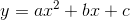is atSo here,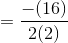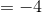We plug this value back into the equation of the parabola, to find the value of the function at this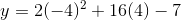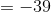Thus the minimal value of the expression is